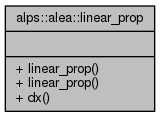ALPSCore reference
alps::alea::linear_prop Struct Reference

#include <propagation.hpp>

Collaboration diagram for alps::alea::linear_prop:## Public Member Functions

linear_prop ()

linear_prop (double dx)

double dx () const

## Detailed Description

Perform linaralized error propagation by estimating the Jacobian.

Given a transformation f and a random sample X, estimate the propagated uncertainties by performing a Taylor series and keeping the linear term:

Cov[f(X)] = df/dX Cov[X] (df/dX)^T + O(d^2f/dx^2)


where df/dX is the Jacobian of f at X, as estimated by finite differences of dx. This procedure is exact for linear transformations; for non-linear transformation, it will introduce bias.

alps::alea::jacobian

Definition at line 43 of file propagation.hpp.

## Constructor & Destructor Documentation

 alps::alea::linear_prop::linear_prop ( )
inline

Definition at line 45 of file propagation.hpp.

 alps::alea::linear_prop::linear_prop ( double dx )
inline

Definition at line 47 of file propagation.hpp.

## Member Function Documentation

 double alps::alea::linear_prop::dx ( ) const
inline

Definition at line 49 of file propagation.hpp.

The documentation for this struct was generated from the following file: